Problem

# CALC In Section 8.5 we calculated the center of mass by considering objects composed...

CALC In Section 8.5 we calculated the center of mass by considering objects composed of a finite number of point masses or objects that, by symmetry, could be represented by a finite number of point masses. For a solid object whose mass distribution does not allow for a simple determination of the center of mass by symmetry, the sums of Eqs. (8.28) must be generalized to integrals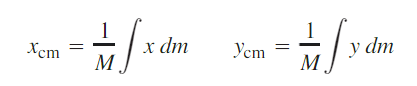where x and y are the coordinates of the small piece of the object that has mass dm. The integration is over the whole of the object. Consider a thin rod of length L, mass M, and cross-sectional area A. Let the origin of the coordinates be at the left end of the rod and the positive x-axis lie along the rod. (a) If the density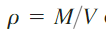of the object is uniform, perform the integration described above to show that the x-coordinate of the center of mass of the rod is at its geometrical center. (b) If the density of the object varies linearly with x—that is,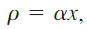where α is a positive constant—calculate the x-coordinate of the rod’s center of mass.

#### Step-by-Step Solution

Solution 1

Solution:

Given data

The length of the thin rod is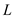.

The mass of the thin rod is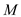Consider a small piece of the rod that has mass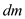and lengthThe cross-sectional area of the thin rod is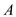.

(a) The density of the thin rod is,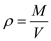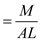Mass of the small piece can be written as,The center of mass of the thin rod has only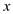coordinate, as the rod lies along theaxis, and it is calculated as,The-coordinate of the center of mass of the thin rod is L/2.

(b) If the density of the object varies linearly with, that is,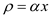Here,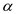is a positive constant

Mass of the small piece can be written as,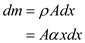The mass of the thin rod is,The-coordinate of the center of mass of the thin rod isAdd your Solution
Textbook Solutions and Answers Search
Solutions For Problems in Chapter 8